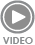# AIR BUOYANCY CORRECTION - 1 Pages

## Catalog excerpts

AIR BUOYANCY CORRECTION new function in analytical balances FUNCTION PERFORMANCE: Function provides two ways of applying the air buoyancy correction. 1. Entering into instrument’s memory determined values of density of the air and density of weighed sample. On specifying these parameters, balance's software automatically calculates the correction factor for measured mass and displays corrected mass value of weight sample. In order to avoid any confusion, the value of corrected mass indication is marked with a special symbol visible on balance’s display, and on a printout. 2.Semi-automatic determining of the air density value by balance’s software and inserting specified value of sample density to instrument's memory. AIM OF FUNCTION: The application enables correcting measuring errors on: 1. Determining mass of substance which density substantially differs from the density of mass standard used for adjusting the balance. As standard, a balance is adjusted using a stainless steel standard with density ~ 8,0 g/cm3 or brass standard with density ~ 8,7 g/cm3. Should any other materials be measured, the following relationship needs considering. Below schema presents the values of correction for mass depending on the density of measured material, assuming that density of the air is constant: 1,2 kg/m3. Density of measured substance A graph of mass corrections depending on density of measured substance Mass of measured sample [g] 2. Testing changes in mass of a sample within a few hours while the sample mass is relatively constant (no substantial changes). It is assumed that the significant impact on the final measurement result is conditioned by on changes in the density of air which is particularly influenced by changes of air pressure, temperature and humidity. Determination of the air density is carried out with use of a set comprising two mass standards, where one of them is made of stainless steel, and the other of aluminum. Weighing and comparing mass indications of the two standards enables automatic calculating the density of air. As the user accepts both mass indications, the software automatically calculates the correction value which is then stored in balance’s memory. Then, the density value of weighed sample should be entered to balance’s memory. On entering the upper mentioned values to software memory, it automatically calculates the corrective factor for weighed mass, and indicates corrected value of sample mass on balance’s display. As in previous case, and in order to avoid any confusion, the value of corrected mass indication is marked with a special symbol visible on balance’s display, and on a printout. The procedure of air buoyancy correction can be switched on and off from the user menu level. The application operates along with other balance’s working modes (checkweighing, filling, etc.). R e l i a b i l i t y o f measurements is conditioned by knowledge of several parameters, including: ambient conditions at the w o r k s t a t i o n , measurement methods, characteristics of tested material, air density in a laboratory room and finally density of tested material.

Open the catalog to page 1